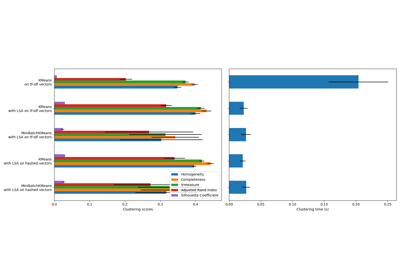# sklearn.feature_extraction.text.TfidfTransformer¶

class sklearn.feature_extraction.text.TfidfTransformer(*, norm='l2', use_idf=True, smooth_idf=True, sublinear_tf=False)[source]

Transform a count matrix to a normalized tf or tf-idf representation

Tf means term-frequency while tf-idf means term-frequency times inverse document-frequency. This is a common term weighting scheme in information retrieval, that has also found good use in document classification.

The goal of using tf-idf instead of the raw frequencies of occurrence of a token in a given document is to scale down the impact of tokens that occur very frequently in a given corpus and that are hence empirically less informative than features that occur in a small fraction of the training corpus.

The formula that is used to compute the tf-idf for a term t of a document d in a document set is tf-idf(t, d) = tf(t, d) * idf(t), and the idf is computed as idf(t) = log [ n / df(t) ] + 1 (if smooth_idf=False), where n is the total number of documents in the document set and df(t) is the document frequency of t; the document frequency is the number of documents in the document set that contain the term t. The effect of adding “1” to the idf in the equation above is that terms with zero idf, i.e., terms that occur in all documents in a training set, will not be entirely ignored. (Note that the idf formula above differs from the standard textbook notation that defines the idf as idf(t) = log [ n / (df(t) + 1) ]).

If smooth_idf=True (the default), the constant “1” is added to the numerator and denominator of the idf as if an extra document was seen containing every term in the collection exactly once, which prevents zero divisions: idf(t) = log [ (1 + n) / (1 + df(t)) ] + 1.

Furthermore, the formulas used to compute tf and idf depend on parameter settings that correspond to the SMART notation used in IR as follows:

Tf is “n” (natural) by default, “l” (logarithmic) when sublinear_tf=True. Idf is “t” when use_idf is given, “n” (none) otherwise. Normalization is “c” (cosine) when norm='l2', “n” (none) when norm=None.

Read more in the User Guide.

Parameters
norm{‘l1’, ‘l2’}, default=’l2’

Each output row will have unit norm, either: * ‘l2’: Sum of squares of vector elements is 1. The cosine similarity between two vectors is their dot product when l2 norm has been applied. * ‘l1’: Sum of absolute values of vector elements is 1. See preprocessing.normalize

use_idfbool, default=True

Enable inverse-document-frequency reweighting.

smooth_idfbool, default=True

Smooth idf weights by adding one to document frequencies, as if an extra document was seen containing every term in the collection exactly once. Prevents zero divisions.

sublinear_tfbool, default=False

Apply sublinear tf scaling, i.e. replace tf with 1 + log(tf).

Attributes
idf_array of shape (n_features)

The inverse document frequency (IDF) vector; only defined if use_idf is True.

New in version 0.20.

References

Yates2011

R. Baeza-Yates and B. Ribeiro-Neto (2011). Modern Information Retrieval. Addison Wesley, pp. 68-74.

MRS2008

C.D. Manning, P. Raghavan and H. Schütze (2008). Introduction to Information Retrieval. Cambridge University Press, pp. 118-120.

Examples

>>> from sklearn.feature_extraction.text import TfidfTransformer
>>> from sklearn.feature_extraction.text import CountVectorizer
>>> from sklearn.pipeline import Pipeline
>>> import numpy as np
>>> corpus = ['this is the first document',
...           'this document is the second document',
...           'and this is the third one',
...           'is this the first document']
>>> vocabulary = ['this', 'document', 'first', 'is', 'second', 'the',
...               'and', 'one']
>>> pipe = Pipeline([('count', CountVectorizer(vocabulary=vocabulary)),
...                  ('tfid', TfidfTransformer())]).fit(corpus)
>>> pipe['count'].transform(corpus).toarray()
array([[1, 1, 1, 1, 0, 1, 0, 0],
[1, 2, 0, 1, 1, 1, 0, 0],
[1, 0, 0, 1, 0, 1, 1, 1],
[1, 1, 1, 1, 0, 1, 0, 0]])
>>> pipe['tfid'].idf_
array([1.        , 1.22314355, 1.51082562, 1.        , 1.91629073,
1.        , 1.91629073, 1.91629073])
>>> pipe.transform(corpus).shape
(4, 8)


Methods

 fit(X[, y]) Learn the idf vector (global term weights). fit_transform(X[, y]) Fit to data, then transform it. get_params([deep]) Get parameters for this estimator. set_params(**params) Set the parameters of this estimator. transform(X[, copy]) Transform a count matrix to a tf or tf-idf representation
__init__(*, norm='l2', use_idf=True, smooth_idf=True, sublinear_tf=False)[source]

Initialize self. See help(type(self)) for accurate signature.

fit(X, y=None)[source]

Learn the idf vector (global term weights).

Parameters
Xsparse matrix of shape n_samples, n_features)

A matrix of term/token counts.

fit_transform(X, y=None, **fit_params)[source]

Fit to data, then transform it.

Fits transformer to X and y with optional parameters fit_params and returns a transformed version of X.

Parameters
X{array-like, sparse matrix, dataframe} of shape (n_samples, n_features)
yndarray of shape (n_samples,), default=None

Target values.

**fit_paramsdict

Returns
X_newndarray array of shape (n_samples, n_features_new)

Transformed array.

get_params(deep=True)[source]

Get parameters for this estimator.

Parameters
deepbool, default=True

If True, will return the parameters for this estimator and contained subobjects that are estimators.

Returns
paramsmapping of string to any

Parameter names mapped to their values.

set_params(**params)[source]

Set the parameters of this estimator.

The method works on simple estimators as well as on nested objects (such as pipelines). The latter have parameters of the form <component>__<parameter> so that it’s possible to update each component of a nested object.

Parameters
**paramsdict

Estimator parameters.

Returns
selfobject

Estimator instance.

transform(X, copy=True)[source]

Transform a count matrix to a tf or tf-idf representation

Parameters
Xsparse matrix of (n_samples, n_features)

a matrix of term/token counts

copybool, default=True

Whether to copy X and operate on the copy or perform in-place operations.

Returns
vectorssparse matrix of shape (n_samples, n_features)

## Examples using sklearn.feature_extraction.text.TfidfTransformer¶